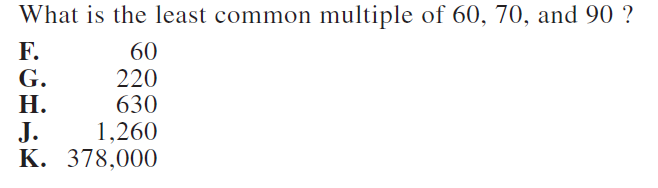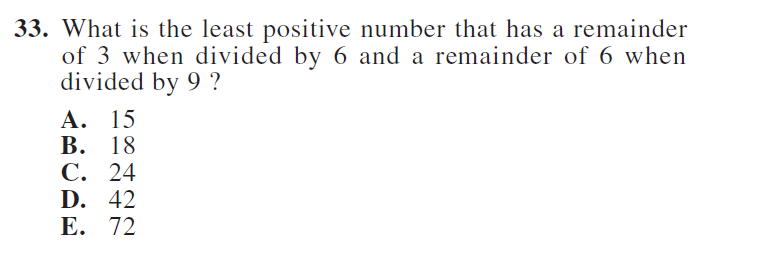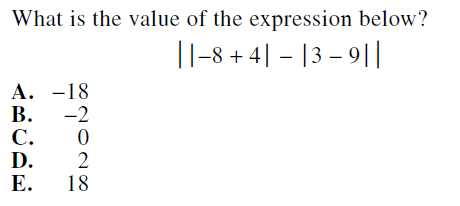## Least Common Multiple (LCM)

To find the least common multiple of a set of numbers you have several methods to choose from.

1. List out the multiples of each number and see which one is the least they have in common.
2. Use the prime factors of each each number to determine the least common multiple mathematically.
3. Use the built in functionality of the TI-84.

Watch this tutorial to learn how to solve LCM ACT Math problems. LCM Tutorial

Example LCM Problem## Greatest Common Factor (GCF)

To find the greatest common factor of a set of numbers you have several methods to choose from.

1. List out the factors of each number and see which one is the greatest they have in common.
2. Use the prime factors of each each number to determine the greatest common factor mathematically.
3. Use the built in functionality of the TI-84.

Watch this tutorial to learn how to solve GCF ACT Math problems. GCF Tutorial

Example GCF Problem## Divisibility

In these types of problems you need to choose the correct number that gives a certain remainder when divided by a particular number.
Skills needed

1. Eliminate answer choices that are divisible by the number.
2. Do long division in order to get the remainder.
3. Useful to know your times tables.

Example Divisibility Problem## Absolute Value

When you see a numuber or expression with a set of vertical bars, that means find the absolute value. The absolute value of a number is how far it is from zero and it always positive. So, simply make what's inside the vertical bars positive.

Example Absolute value Problem